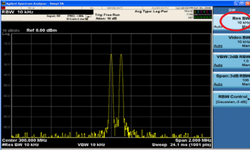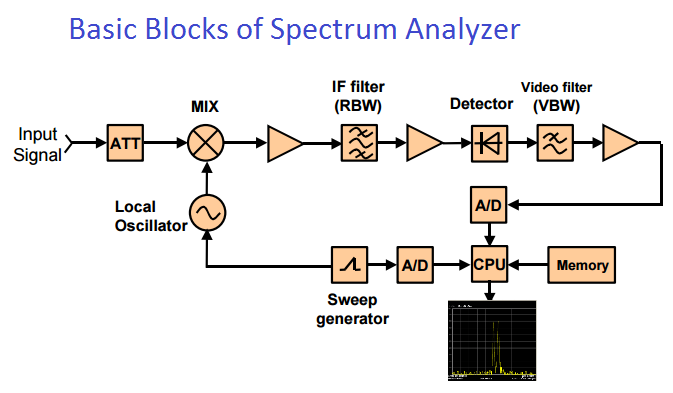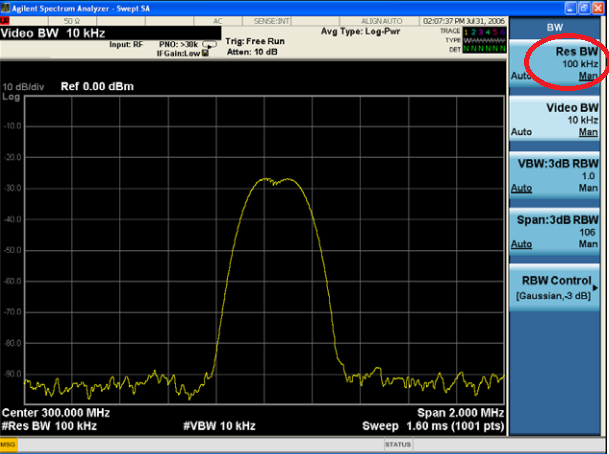# What is RBW of instrument, why it is important for signal analysis ?

RBW stands for Resolution Bandwidth; it is used to view distinctly two very closely spaced signals in frequency domain.Two input signals can be seen as two spectrum waveforms only if they exceed the 3dB bandwidth of the IF filter. Typically, resolution bandwidth is defined as the 3 dB bandwidth of the IF filter. However,resolution bandwidth may also be defined as the 6 dB or impulse bandwidth of the filter

These filters are bandpass filters located in the spectrum analyser’s final IF stages.

### Resolving Closely Spaced Signals

As you change the filter bandwidth, you change the width of the displayed response. If a wide filter is used and two equal-amplitude input signals are close enough in frequency, then the two signals will appear as one signal. If a narrow enough filter is used, the two input signals can be discriminated and appear as separate peaks. Thus, signal resolution is determined by the IF filters inside the analyzer.

Below is the example of two closely spaced signals:

RBW is 100KHzRBW is 10KHz### RBW affecting Noise Floor

Lowering the RBW lowers down the noise floor of the instrument. So if you want to analyze the very low value signal, then in that case, RBW needs to be lower down accordingly.